# High School Math : Rhombuses

## Example Questions

### Example Question #1 : How To Find If Rhombuses Are Similar

A quadrilateral ABCD has diagonals that are perpendicular bisectors of one another. Which of the following classifications must apply to quadrilateral ABCD?

I. parallelogram

II. rhombus

III. square

I and II only

I, II, and III

I and III only

II and III only

I and II only

Explanation:

If the diagonals of a quadrilateral are perpendicular bisectors of one another, then the quadrilateral must be a rhombus, but not necessarily a square. Since all rhombi are also parallelograms, quadrilateral ABCD must be both a rhombus and parallelogram.

### Example Question #677 : High School Math

This figure is a rhombus with a side of 8 in.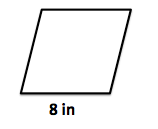What is the area of the rhombus (in2)?

Not enough information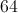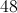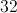Not enough information

Explanation:

In order to determine the area of a rhombus you either need a base and a height OR the length of the two diagonals OR the base and an angle.  Because we are only given the base with no information about the height, there is not enough information to determine the area of the rhombus.

### Example Question #678 : High School Math

Find the area of the following rhombus: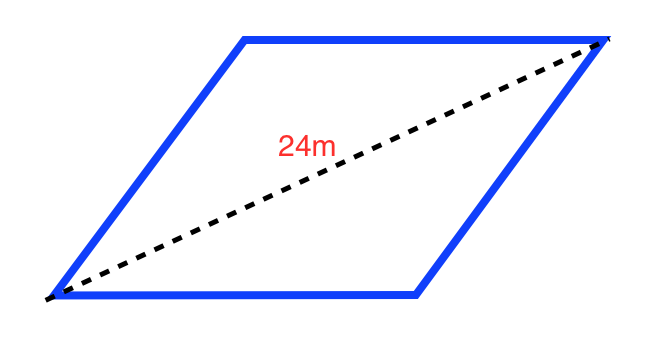The perimeter of the rhombus is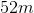.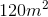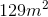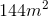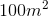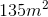Explanation:

The formula for the perimeter of a rhombus is: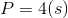Whereis the length of the side

Plugging in our values, we get: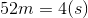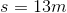The formula for the area of a rhombus is: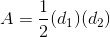Where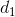is the length of one diagonal and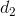is the length of another diagonal

By drawing the diagonals, we create a right triangle with the hypotenuse as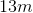and the side as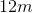.

Since we know that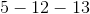is a phythagorean triple, we can infer that the third side is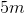.

Plugging in our values, we get: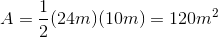### Example Question #679 : High School Math

This figure is a rhombus with a side of 8 in.What is the perimeter of the rhombus (in)?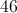Explanation:

The perimeter of the rhombus is the distance around the outside of the figure.  Since a rhombus has 4 congruent sides, the perimeter is simply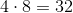.

### Example Question #680 : High School Math

You are given a rhombus with a side ofmeters. What is the perimeter of the rhombus?metersmetersmetersmetersmetersmeters
A rhombus is a quadrilateral (sided figure) withcongruent sides. The perimeter is the sum of the sides. Thus, to find the perimeter of the rhombus, we multiply the perimeter by. This gives usmeters.